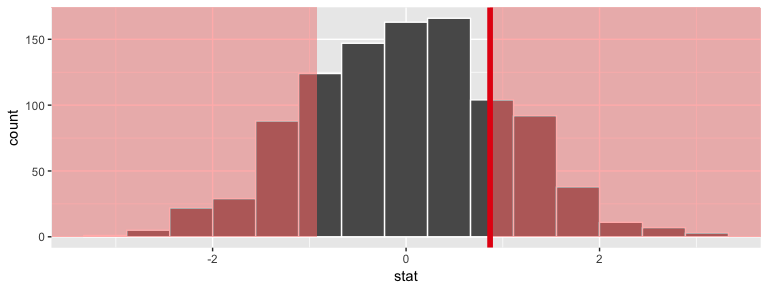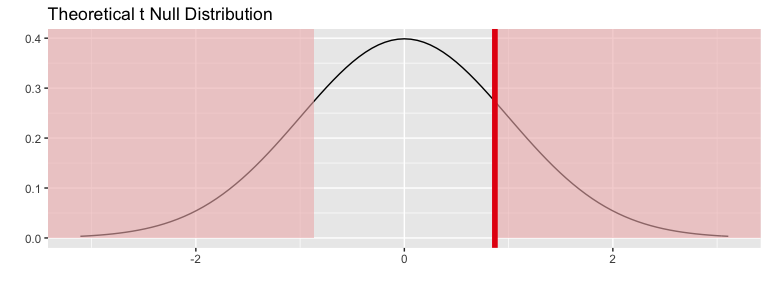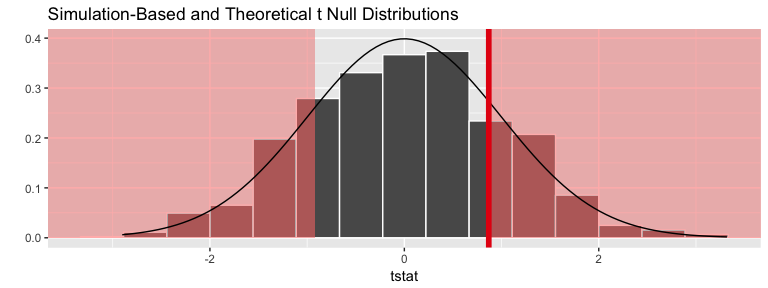# Two sample $$t$$ test example using nycflights13flights data

## Data preparation

library(nycflights13)
library(dplyr)
library(stringr)
library(infer)
set.seed(2017)
fli_small <- flights %>%
sample_n(size = 500) %>%
mutate(half_year = case_when(
between(month, 1, 6) ~ "h1",
between(month, 7, 12) ~ "h2"
)) %>%
mutate(day_hour = case_when(
between(hour, 1, 12) ~ "morning",
between(hour, 13, 24) ~ "not morning"
)) %>%
select(arr_delay, dep_delay, half_year,
day_hour, origin, carrier)
• Two numeric - arr_delay, dep_delay
• Two categories
• half_year ("h1", "h2"),
• day_hour ("morning", "not morning")
• Three categories - origin ("EWR", "JFK", "LGA")
• Sixteen categories - carrier

# One numerical variable, one categorical (2 levels)

## Calculate observed statistic

Using t_test in infer

obs_t <- fli_small %>%
t_test(formula = arr_delay ~ half_year) %>%
dplyr::select(statistic) %>%
dplyr::pull()

The observed $$t$$ statistic is 0.8685463.

Or using another shortcut function in infer:

obs_t <- fli_small %>%
t_stat(formula = arr_delay ~ half_year)

The observed $$t$$ statistic is 0.8685463.

## Randomization approach to t-statistic

t_null_distn <- fli_small %>%
# alt: response = arr_delay, explanatory = half_year
specify(arr_delay ~ half_year) %>%
hypothesize(null = "independence") %>%
generate(reps = 1000, type = "permute") %>%
calculate(stat = "t", order = c("h1", "h2"))
## Warning: Removed 15 rows containing missing values.
t_null_distn %>% visualize(obs_stat = obs_t, direction = "two_sided")## Calculate the randomization-based $$p$$-value

t_null_distn %>%
dplyr::summarize(p_value = mean(abs(stat) >= obs_t)) %>%
dplyr::pull()
##  0.42

## Theoretical distribution

fli_small %>%
# alt: response = arr_delay, explanatory = half_year
specify(arr_delay ~ half_year) %>%
hypothesize(null = "independence") %>%
# generate() ## Not used for theoretical
calculate(stat = "t", order = c("h1", "h2")) %>%
visualize(method = "theoretical", obs_stat = obs_t, direction = "two_sided")
## Warning: Removed 15 rows containing missing values.
## Warning: Check to make sure the conditions have been met for the
## theoretical method. infer currently does not check these for you.## Overlay appropriate $$t$$ distribution on top of permuted t-statistics

fli_small %>%
# alt: response = arr_delay, explanatory = half_year
specify(arr_delay ~ half_year) %>%
hypothesize(null = "independence") %>%
generate(reps = 1000, type = "permute") %>%
calculate(stat = "t", order = c("h1", "h2")) %>%
visualize(method = "both", obs_stat = obs_t, direction = "two_sided")
## Warning: Check to make sure the conditions have been met for the
## theoretical method. infer currently does not check these for you.## Compute theoretical p-value

fli_small %>%
t_test(formula = arr_delay ~ half_year) %>%
dplyr::select(p_value) %>%
dplyr::pull()
##  0.3855325